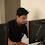# HackerRank Lower Bound-STL solution in c++ programming

In this HackerRank Lower Bound-STL problem in c++ programming language, You are given N integers in sorted order. Also, you are given Q queries. In each query, you will be given an integer and you have to tell whether that integer is present in the array. If so, you have to tell at which index it is present and if it is not present, you have to tell the index at which the smallest integer that is just greater than the given number is present.

## HackerRank Lower Bound-STL problem solution in c++ programming.

```#include <cmath>
#include <cstdio>
#include <vector>
#include <iostream>
#include <algorithm>
using namespace std;

int main() {
/* Enter your code here. Read input from STDIN. Print output to STDOUT */
int n;
vector<int> vec;
cin >> n;
for (int i = 0; i < n; i++) {
int temp;
cin >> temp;
vec.push_back(temp);
}
int q;
cin >> q;
for (int i = 0; i < q; i++) {
int temp;
cin >> temp;
vector<int>::iterator iter;
iter = lower_bound(vec.begin(), vec.end(), temp);
if (*(iter + 1) == temp || *iter == temp)
cout << "Yes " << iter - vec.begin() + 1 << endl;
else
cout << "No " << iter - vec.begin() + 1 << endl;
}
return 0;
}```

## Second solution

```#include <cmath>
#include <cstdio>
#include <vector>
#include <iostream>
#include <algorithm>
using namespace std;

int main() {
int n;
vector<int> data;
cin >> n;
while (n--) {
int x;
cin >> x;
data.push_back(x);
}

cin >> n;
while (n--) {
int x;
cin >> x;
int index = lower_bound (data.begin(), data.end(), x) - data.begin();
if (data[index] == x)
cout << "Yes ";
else
cout << "No ";

cout << index + 1 << endl;
}
return 0;
}```

1.2.